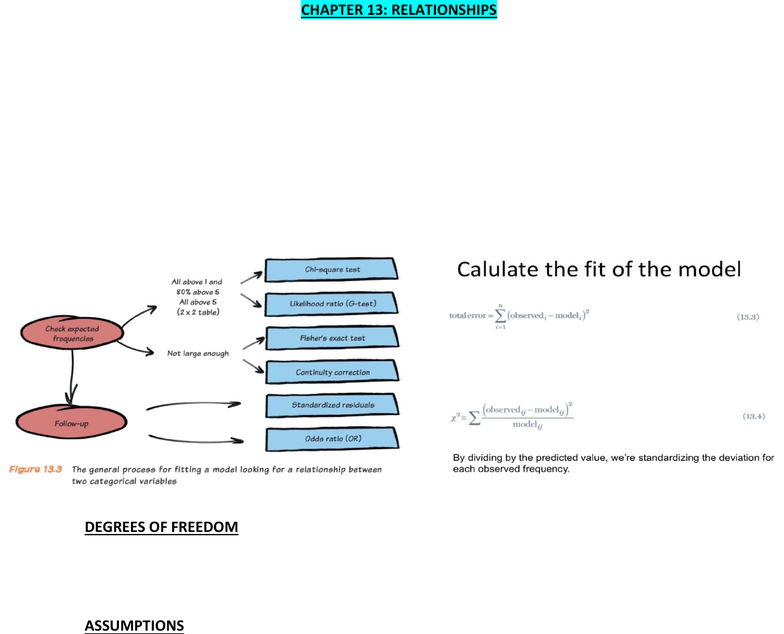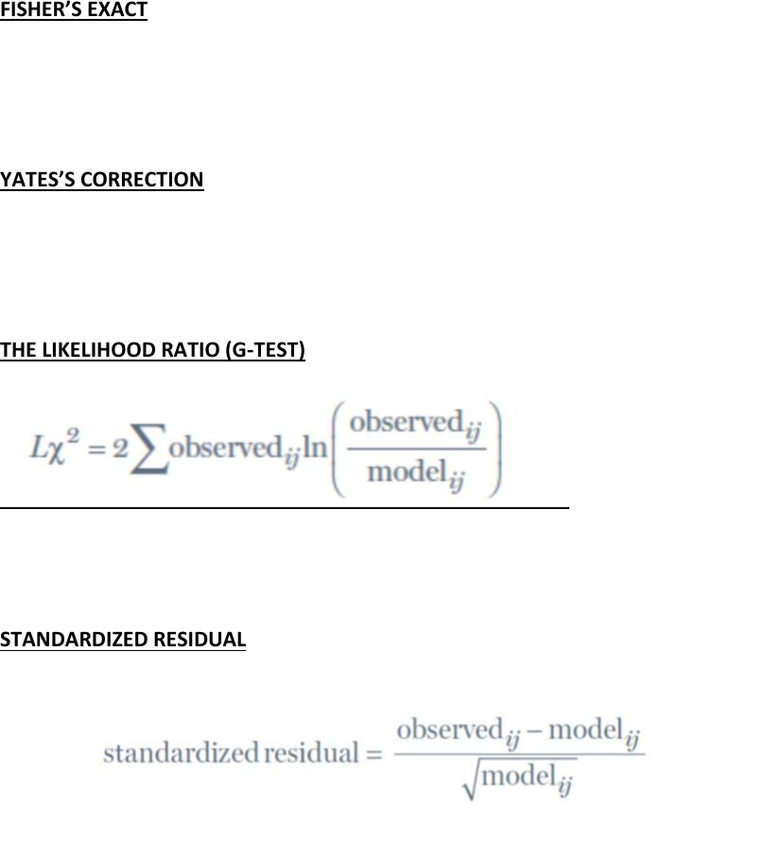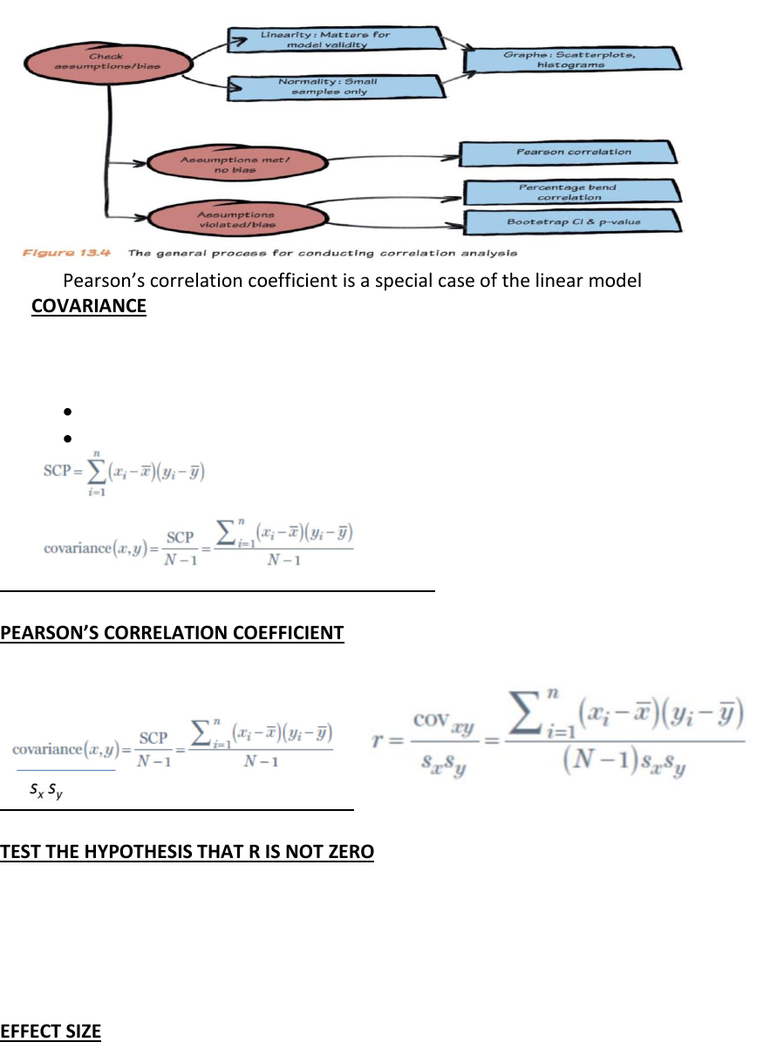Textbook Notes (280,000)
CA (170,000)
McMaster (10,000)
PNB (70)
Chapter 13-15

# PNB 3XE3 Chapter Notes - Chapter 13-15: Likelihood Ratios In Diagnostic Testing, Categorical Variable, Effect Size

Department
Psychology, Neuroscience & Behaviour
Course Code
PNB 3XE3
Professor
Rutherford
Chapter
13-15

This preview shows pages 1-3. to view the full 11 pages of the document.CHAPTER 13: RELATIONSHIPS
THINGS TO KNOW
Chi-square test: Finding relationships in categorical data
o How to do it
Calculate expected values
Calculate Chi-square (this is your test statistic)
Assumptions
Fisher’s exact test
The likelihood ratio
Standardized residuals
Effect size
DEGREES OF FREEDOM
The degrees of freedom are calculated as (r-1)(c-1), in which r is the number of rows and
c is the number of columns
*There is a practice example in the chapter 13 slides!
ASSUMPTIONS
Independent errors
o Each case (entity) contributes to only one cell
Sample size
o If 2x2, no expected frequency less than 5
o If larger
No more than 20% of cells less than 5
All expected frequencies greater than 1
Else use Fisher’s exact

Only pages 1-3 are available for preview. Some parts have been intentionally blurred.FISHER’S EXACT
Computes the probability of your X2 value
o Each case (entity) contributes to only one cell
Use it with a 2x2 table
Usually just with a small sample size
YATES’S CORRECTION
Shaves 0.5 off of each cell difference before you sum together to find X2.
o Each case (entity) contributes to only one cell
Makes X2 smaller and p bigger
More conservative
THE LIKELIHOOD RATIO (G-TEST)
The test statistic has a chi-square distribution
It is preferred when samples are small
It will be the same as X2 when samples are large
STANDARDIZED RESIDUAL
Tells you what is contributing to the X2 value that you calculated
They are z-scores
o Z-score with a value outside of +/- 1.96 will be significant at p < 0.05
o Z-scores with a value outside of +/- 2.58 is significant at p <0.01

Only pages 1-3 are available for preview. Some parts have been intentionally blurred.- Pearson’s correlation coefficient is a special case of the linear model
COVARIANCE
If two variables are related, then if an observation is far from the mean on one
variable, it should be far from the mean on the other variable
Multiply the deviation for one variable by the corresponding deviation for the other
This captures the positive or negative nature of the relationship
Sum of cross-product deviations
PEARSON’S CORRELATION COEFFICIENT
TEST THE HYPOTHESIS THAT R IS NOT ZERO
Pearson’s r does not have a normal sampling distribution, but it can be transformed so
that it does
o Use a z score zr. it is normally distributed, it has a known standard error
o Or use a t statistic tr.
Changes shape with the sample size
Look it up in a t-table or use SPSS
EFFECT SIZE
R is an effect size
R2 is a measure of the amount of variability in one variable that is shared by the other